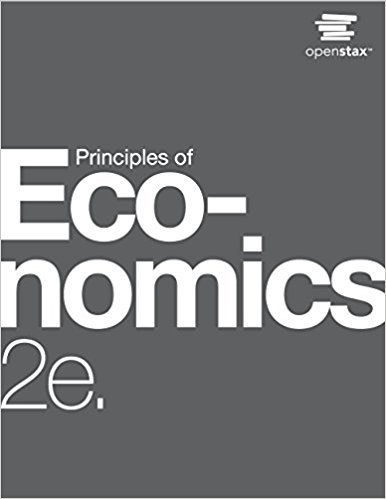×
Get Full Access to Principles Of Economics - 2 Edition - Chapter 34 - Problem 55
Get Full Access to Principles Of Economics - 2 Edition - Chapter 34 - Problem 55

×

# Solved: Assume two countries, Thailand (T) and Japan (J), have one good: cameras. TheISBN: 9781947172364 471

## Solution for problem 55 Chapter 34

Principles of Economics | 2nd Edition

• Textbook Solutions
• 2901 Step-by-step solutions solved by professors and subject experts
• Get 24/7 help from StudySoup virtual teaching assistantsPrinciples of Economics | 2nd Edition

4 5 1 336 Reviews
25
2
Problem 55

Assume two countries, Thailand (T) and Japan (J), have one good: cameras. The demand (d) and supply (s) for cameras in Thailand and Japan is described by the following functions: QdT = 60 P QsT = 5 + 1 4 P QdJ = 80 P QsJ = 10 + 1 2 P P is the price measured in a common currency used in both countries, such as the Thai Baht. a. Compute the equilibrium price (P) and quantities (Q) in each country without trade. b. Now assume that free trade occurs. The freetrade price goes to 56.36 Baht. Who exports and imports cameras and in what quantities?

Step-by-Step Solution:
Step 1 of 3

Drug and Alcohol Abuse: Chapter 1 1. What constitutes a drug a. Any substance that modifies (enhances, inhibits, or distorts) mind/body functioning b. What are the psychoactive drugs i. Drug compounds (substances) that affect the central nervous system and/or alter consciousness and/or perceptions ii. Classified as either 1. Licit (legal): coffee, tea, alcohol, tobacco, over the counter drugs…

Step 2 of 3

Step 3 of 3

##### ISBN: 9781947172364

The answer to “Assume two countries, Thailand (T) and Japan (J), have one good: cameras. The demand (d) and supply (s) for cameras in Thailand and Japan is described by the following functions: QdT = 60 P QsT = 5 + 1 4 P QdJ = 80 P QsJ = 10 + 1 2 P P is the price measured in a common currency used in both countries, such as the Thai Baht. a. Compute the equilibrium price (P) and quantities (Q) in each country without trade. b. Now assume that free trade occurs. The freetrade price goes to 56.36 Baht. Who exports and imports cameras and in what quantities?” is broken down into a number of easy to follow steps, and 107 words. Principles of Economics was written by and is associated to the ISBN: 9781947172364. Since the solution to 55 from 34 chapter was answered, more than 231 students have viewed the full step-by-step answer. The full step-by-step solution to problem: 55 from chapter: 34 was answered by , our top Business solution expert on 03/16/18, 04:24PM. This full solution covers the following key subjects: . This expansive textbook survival guide covers 37 chapters, and 1291 solutions. This textbook survival guide was created for the textbook: Principles of Economics, edition: 2.

Unlock Textbook Solution